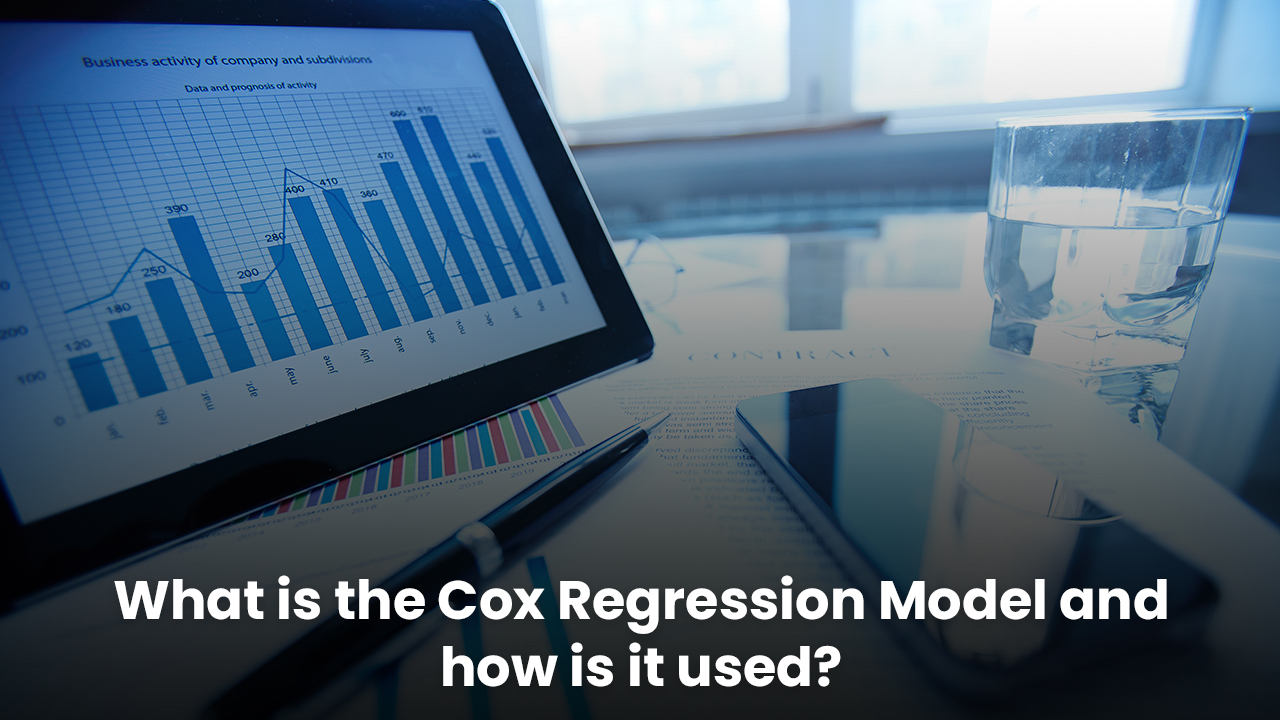# What is the Cox Regression Model and how is it used?## Introduction

The Cox Regression or the Proportional Hazards Regression is a method conducted with SPSS help performs investigating activities with the effect of various variables depending upon the time in which a specified event is going to take place in action. Once this Cox model is built fully from the observed and collected values, then it will be used for making different predictions with the new data inputs. The Cox Regression model was generally drawn up as a predictive model for analysing the time-to-event data. Cox regression analysis is a technique for assessing the association between variables and survival rate.

## Cox Regression Model

The Cox's Proportional Hazards regression Model which is known also by the name of Cox Regression or by Cox's Model introduced in the year 1972, basically builds a form of survival analysis functions, all of which tells the probability of a certain event for example - a death, if happens at a fixed particular time. This model is essentially such a regression model that can be commonly used as a statistical spss cox regression in conducting the medical research work, investigating along with the association in between the survival time of the patients and among one or more predictor variables. This model is basically a type of survival analysis regression model in which there is a description of the relationships of the event incidence, which is expressed by the set of hazardous functions along with a set of variety of covariates. The multivariate analysis by applying the method of SPSS Cox Regression is then implemented when there is the need for multiple and potentially interacting covariates. In recent years, experts use SPSS data analysis for multivariate survival analysis with the use of the Cox's regression model which has been utilised increasingly for conducting the analysis of the censored survival of data.## Use of Cox Regression Model

The model of Cox Proportional Hazards is typically a Semi-Parametric Model since there exist no clear assumptions regarding the shape of this baseline of hazard functions. There are several Cox models, but the best Cox models are particularly those which include the censored data and observations where events didn’t expect to happen as well as the collected data from various observations where events have actually occurred. There are some of the other regression models to all of which are used in the survival analysis by the SPSS experts in assuming the specific distributions of all the survival times like the Weibull, Gompertz, exponential and other log-normal distributions. The Cox regression can too handle all the quantitative predictor variables and also the categorical variables. It also tackles those problems which are related to participant heterogeneity. Though, it is much popular in the process of survival analysis that the Cox regression has its downside which is compared with all the other methods of regression which can be much difficult to understand. Various types of technical computations are required in this process which includes the copious matrix multiplications and inversions too. And this characteristic makes it extremely challenging to calculate by only using hand, but there exist many other statistical packages which is quite responsible for handling the particular type of Cox regression. The few steps of using the Cox Regression Model are like:

1. Step 1: Click on the “Analyse” option–go to the “Survival” option–select the Cox Regression
2. Step 2: Then choose a particular time variable except the negative time values since this analysis will automatically exclude the negative time values
3. Step 3: After that select the significant status variable
4. Step 4: Then click on the “Define Event”
5. Step 5:Then it’s time to choose the covariates.

Optional Note: it is required to select any such variables which are interacting and then click on the

## Conclusion

The Cox's Proportional Hazards regression model which is known as Cox Regression or by Cox's Model introduced in the year 1972, is essentially such a regression model that is used as a statistical SPSS cox regression investigating along with the association in between the survival time of the patients and among one or more predictor variables. The multivariate analysis by applying the method of SPSS Cox Regression is then implemented when there is the need for multiple and potentially interacting covariates. There are several Cox models, but the best are those which include the censored data and observations where events didn’t happen as well as the collected data from various observations where events have actually happened

Roman Thomson - Tue, 20 Sep 2022

I was struggling to understand cox regression and this article helped me clarify my doubts. Thanks to you. The steps you've mentioned are just amazing, I needed something like that.

Hector Cox - Mon, 20 Jun 2022

Nobody was able to make me understand the cox regression model, but this article did. Well understood, Thanks.

Helly Max - Tue, 31 May 2022

An engaging article, especially the steps mentioned here. These steps helped me perform my data analysis.

Leesa Flores - Tue, 24 May 2022

Very impressive and amazingly written essay.